# Surface and interfacial tension

If a small quantity of liquid is in equilibrium with its vapor, it will spontaneously assume a spherical form. This is also true for drops suspended in a liquid with which they are immiscible. The surface or Interface is in a state of tension and work would have to be done to extend the surface or interface. Thus there is an excess Free Energy associated with any system which contains a surface or interface. This free energy, σ may be defined as the reversible work done to increase the surface or interface by unit area isothermally. The units are in J m−2 and the new surface or interface has identical properties to the original.
The surface is in a state of tension. The tension, however, does not increase as the surface is extended but remains constant at a value characteristic of the liquid under those conditions. It is a real force, which can be used to support mass as, for example, in the flotation of heavy mineral ores where the particles of ore are brought to the surface by attachment to small bubbles rising through the liquid. The surface tension, γ, may be defined as the force/unit length parallel to the surface which is exerted perpendicular to any line drawn in the surface. Surface tension is sensitive to curvature of the surface only for very small radii of curvature (see interfaces). The units of surface tension are mNm−1. The same arguments hold for the interface between two pure immiscible liquids. For such interfaces and for pure liquids in equilibrium with their vapor the excess free energy, σ, and the surface or interfacial tension, γ, are numerically equal and have the dimensions (MT−2). In this work the symbol for both of these quantities will be σ.
An excess surface free energy is also associated with a solid in equilibrium with its vapor. Since the atoms or molecules in a solid have limited mobility a freshly formed solid surface is rarely in true equilibrium. The values of σ for solids are very large compared with those for liquids and are difficult to measure. Calculated values exist for many ionic crystals and cleavage and fracture methods of measurement have been used for single crystals of mica and for alkali halides at low temperatures where brittle fracture takes place. The surface tension for metals has been measured for pure metals by observing the extension and contraction of wires with temperature when they are hung outside evacuated crucibles made of the same metal as the wires and weights. Typical values of the surface tensions of some solids are shown in Table 1.

Table 1. Surface energy values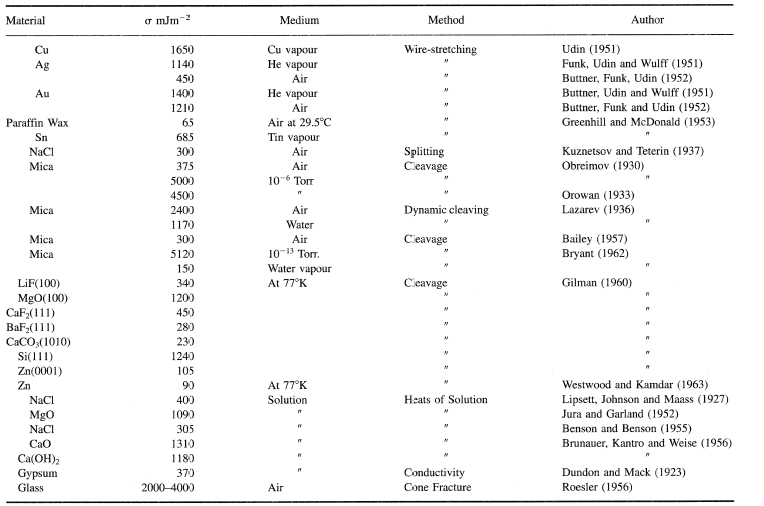Bailey, A. I. (1957) Proc. 2nd Int. Cong. Surf. Act., Ill, 189.
Bailey, A. I. (1957) Ibid. 406.
Benson, G. C. and Benson, G. E. (1955) Can. J. Chem., 33, 232.
Brunauer, S., Kantro and Weise (1956) Can. J. Chem., 34, 729.
Bryant. P. J. (1962) Trans. Ninth Vac. Symp., U.S.A..
Buttner, F., Udin, H., and Wulff, J. (1951) Ibid. 1209.
Dundon, M. L. and Mack, E. (1923) J. Amer. Chem. Soc., 45, 2479.
Funk, E. R., Udin, H., and Wulff, J. (1951) J. Metals, 3, 1206.
Buttner, F. H., Funk, E. R., and Udin, H. (1952) J. Phys. Chem., 56, 657.
Gilman, J. J. (1960) J. Appl. Phys., 36, 1374.
Greenhill, E. B. and McDonald, S. R. (1953) Nature, 171, 37.
Jura, G. and Garland, C. W. )(1952) J. Amer. Chem. Soc., 74, 6033.
Kuznetsov, V. D. and Teterin. P. P. (1937) The Physics of Solids by Kuznetsov, Vol. I, 388.
Lazarev, V. P. (1936) Zhur. Fiz. Khim., 7, 320.
Lipsett, S. G., Johnson, F. M. G., and Maass, O. (1927) J. Amer. Chem. Soc., 49, 925.
Obreimov, I. V. (1930) Proc. Roy. Soc., A127, 290.
Orawan, E. (1933) Zeit. f. Phys., 82, 235.
Udin, H., Shaler, A. J., and Wulff, J. (1949) J. Metals, 1, 186.
Udin, H. (1951) J. Metals, 3, 63
Roesler, F. C. (1956) Proc. Phys. Soc., B69, 981.
Westwood, A. R. C. and Kamdar, M. H. (1963). Phil. Mag., 8, 7, 87.

### Molecular Theory of Interfacial Tension

The interface between two immiscible liquids is in a state of tension in a manner analogous to the liquid-vapor interface. This can be seen if droplets of one liquid are dropped into the bulk of another liquid of nearly equal density. The droplets assume a spherical shape. The molecules in the interfacial region are at a higher potential than those in the corresponding bulk. Girifalco and Good (1957) were the first to show how the interfacial tension could be expressed in terms of the intermolecular forces operating in the system. Three sets of forces must be considered when liquid one is in contact with liquid two. These are the forces acting between like molecules in liquid one and in liquid two plus those which act across the interface between molecules of liquids one and two.
These forces were expressed in terms of the surface tensions of the two liquids. The work of adhesion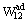was then given by
(1)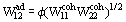where
(2)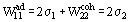and
(3)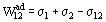φ is a constant characteristic of the system. Hence,
(4)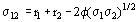A further contribution was made by Fowkes who assumed that the contributions made by different types of intermolecular interaction are additive and could be assessed separately. Hence the surface tension of a liquid would consist of two components, σd, due to dispersion forces and σp due to polar interactions which include Keeson and Debye forces as well as hydrogen bonding. Pure hydrocarbon liquids interact with other liquids through dispersion forces only and the interfacial tension between liquids, a hydrocarbon and liquid two is then given by
(5)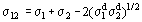The factor two allows for dispersion interactions between both liquids one and two and two and one. In addition he realized that if one partner in the pair of liquids acted as a Lewis acid while the other acted as a Lewis base, the interfacial tension between the two would be further reduced [Fowkes (1972)]. The effect on the interfacial tension of changing the pH of the aqueous phase will indicate whether the water-immiscible organic liquid acts as a Lewis acid or a Lewis base. In the former case an increase of pH causes a lowering of the interfacial tension while the opposite is true in the case of a Lewis base when a lowering of the interfacial tension accompanies a decrease in pH (Table 2).

Table 2. Values of σL and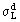for a number of liquids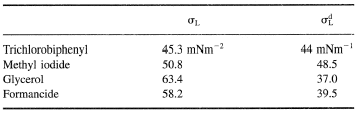Just as the dispersion and polar contribution to the surface tension of liquids may be determined by combining the liquids with pure hydrocarbons, so the method may be extended to solid-liquid interfaces, thus from Young's equation.
(6)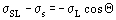(7)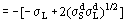(8)This relation has been tested experimentally and the results shown in Figure 1. The contact angles formed by a number of liquids on the solid are measured,is determined from interfacial tension measurements using pure hydrocarbons. Hence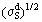can be obtained from the slope of the lines obtained by plotting Θ against ()1/2L.  Dann (1970) expressed the polar contribution to the interfacial tension in terms of the spreading coefficient, s, which can be measured
(9)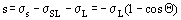If dispersion forces alone act we may calculate the spreading coefficient, sd, from
(10)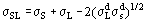giving
(11)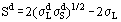(12)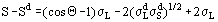(13)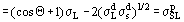Figure 2a and b shows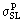plotted againstfor paraffin wax in which the only interactions would be dispersion interactions and for polystyrene where polar interactions would be expected. The results confirm that for paraffin wax the interaction is constant no matter how polar the liquid. The methods provide means by which the polar and dispersion contributions for the interaction between liquids and solids may be determined.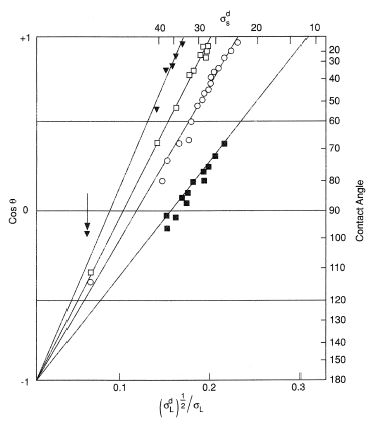Figure 1. Contact angle of a number of liquids on low energy surfaces.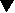: polyethylene;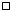: paraffin wax;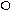: C36H74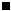: fluorodecanoic acid monolayer on platinum. All contact angles below the arrow are with water.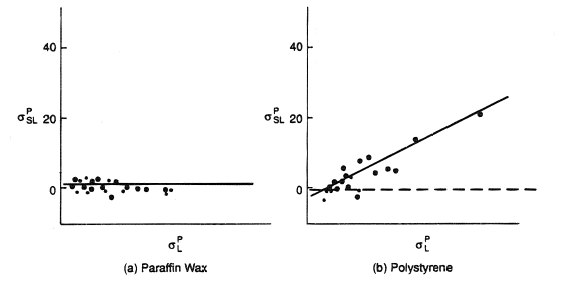Figure 2.

### Methods of Measuring Interfacial Tension

#### Capillary rise method

The difference in pressure across a curved meniscus results in the phenomenon of capillarity. A liquid which meets the surface of a fine pore or tube will rise in it if the end of the tube dips into a pool of the liquid. Conversely a liquid which makes a Contact Angle which is greater than 90° will experience a depression. This phenomenon provides a simple and accurate technique for the measurement of the surface tension of a liquid as shown in Figure 3.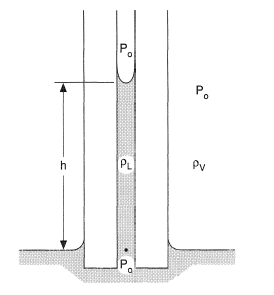Figure 3. Capillary rise method.
If a tube, which is wet by a liquid is dipped into it, liquid rises up the tube to a height h. This is due to the Laplace pressure difference across the curved meniscus formed in the capillary. Equating pressures, we have
(14)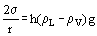where r is the radius of the tube; ρL the density of the liquid; and ρv the density of the vapor.
If the liquid forms a finite contact angle, Θ, with the tube, then
(15)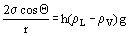The method is only accurate for Θ = 0.
If the radius of tube is appreciable, then r is replaced by b where b refers to the radius of curvature of the liquid, and a relation between b and r must be found. The tube must be of uniform bore and the height h must be measured from the flat surface in the reservoir. If sufficient liquid to fill a large reservoir is not available, a differential method using two capillaries of different bore may be used, thus
(16)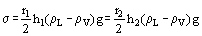or
(17)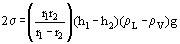For samples of very small volume Fergusons' modification of the capillary rise may be used as shown in Figure 4. Here the pressure required to force a meniscus located at the end of a capillary into planar form is measured. Θ must be zero.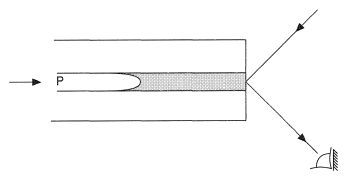Figure 4. Fergusons' modified method.

#### The drop volume method

This is one of the commonest and most convenient.
The volume or weight of drops, which form slowly from the tip of a vertical tube is measured. From Figure 5 the upward force balancing the drop gives 2πrσ = mg. This is an oversimplification. Figure 6 shows the sequence of processes involved as a drop detaches from the top. Finally, two drops, one large and one small, break away and both are measured. Some liquid is retained on the tip. Careful work shows that the weight of drops is a function of r/V1/3, where r is the radius of the tube and V is the volume of drops. Hence
(18)or
(19)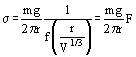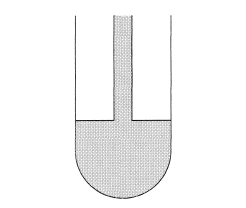Figure 5. Drop volume method.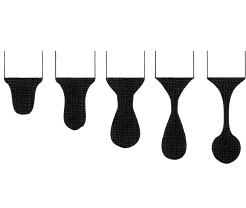Figure 6. Drop detatchment sequence.
Correction tables giving F for values of r/V1/3 have been made. The form of the correction is shown in Figure 7. The accuracy of the method is 0.1%, where for pure non-volatile liquids it is 0.02%. The weight or volume of a number of drops is determined. The tip of tube must be ground flat and r measured. The final stages of formation of each drop must take place slowly. If the reservoir is adjustable in the vertical direction, the drop may be formed initially using a relatively large head, which is later reduced. The drop must be in equilibrium with the vapor.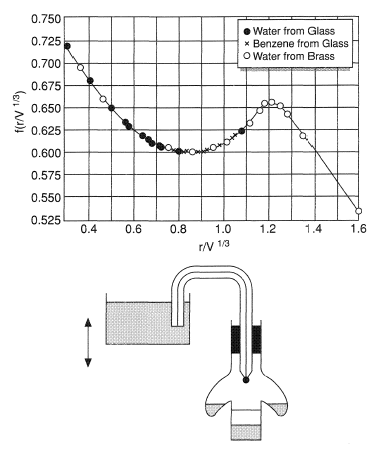Figure 7. Correction Factor, F.

#### Maximum bubble pressure method

A bubble is blown at the tip of a tube dipping in a liquid. As the bubble grows the radius of curvature of the interface decreases at first, reaches a minimum and then increases again, as shown in Figure 8.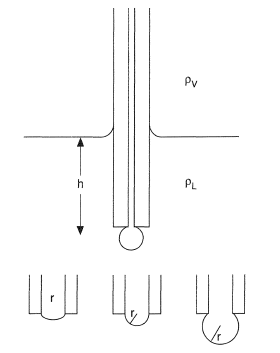Figure 8. Maximum bubble pressure method.
At maximum pressure
(20)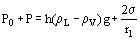where Po = h(ρL − σV)g is the part of the pressure required to force the liquid down the tube to a depth h. Since the surface of the liquid in the reservoir must be flat the experiment is usually carried out as a differential method using tubes of different bore at the same depth in the liquid.
(21)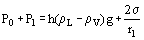(22)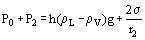therefore
(23)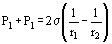The tip of the tube must be ground flat and the capillary diameter should be as small as possible.
The method has an accuracy of about 0.5% and can be used for interfacial tensions between liquids.

### The Shapes of Sessile and Pendant Drops and Bubbles

The fundamental equation for the surface separation between two fluids is
(24)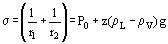where Po is the pressure at the apex, 0, of the drop or bubble at which point r1 = r2 and z is the vertical height of the surface from 0. z is measured positive towards the concave side of the interface.
For any point S in Figure 9 draw SC perpendicular to the interface cutting the axis at C.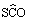= φ. Let the radius of curvature in the plane of the paper at S = r1 = R. And the radius of curvature at right angles to this is r2 where
(25)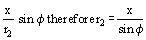therefore
(26)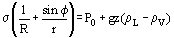(27)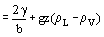where b is the radius of curvature at 0. Hence,
(28)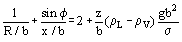Put
(29)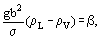then
(30)b is the parameter which determines the scale of the meniscus while β is the parameter which determines the shape of the meniscus, β is positive if the fluid above the interface is lighter and negative if the fluid above the interface is denser.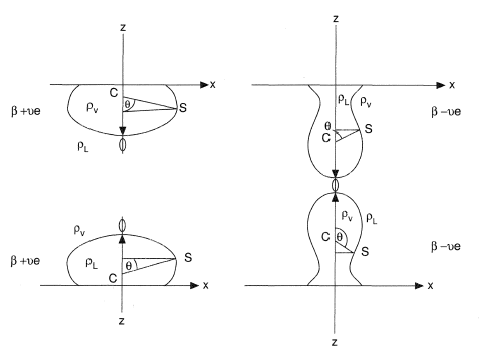Figure 9. Sessile and pendant drops and bubbles.
This equation has been solved numerically. Tables exist giving x/b, z/b, and V/b3, for given values of φ and β. Here V is the volume of the drop. [See Bashforth and Adams (1883), see also Drops.]

#### Sessile drops and bubbles

Results are found to be independent of the contact angle. The method is static and therefore independent of viscosity.
The drops or bubbles are photographed and measured accurately. If the drop is large enough to make the curvature at 0 negligible the only measurements required are the diameter of the equatorial plane and the height, H, from this plane to the apex.
Then
(31)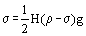Correction tables for H/re, where re is the equatorial radius, are given for cases where the curvature at 0 is not negligible [Fordham (1948)].

#### Pendant drops and bubbles

Drops are formed on the tip of a tube and photographed.
The maximum horizontal diameter, de, of the drop is measured and the width, ds, at a height, de, from the apex as illustrated in Figure 10. We may define:
(32)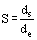and
(33)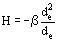(34)Tables of 1/H for values of S have been compiled [Fordham (1948)]. The drop must be protected from vibrations and de must be measured accurately to avoid large errors in ds.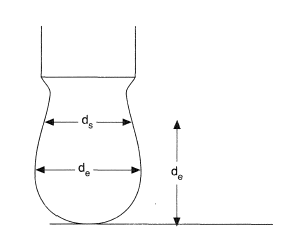Figure 10. Pendant drops and bubbles.

### Detachment Methods du Noüy ring, plate

Surface tension may be determined by measuring the force necessary to detach objects of known shape from the surface. As the object is lifted it lifts a film of fluid with it, which at a certain height becomes unstable and breaks away. Stages of detachment are seen in Figure 11 from which it is evident that R is in error because the meniscus becomes concave before instability occurs, and that some fluid remains attached to the ring after detachment, so
(35)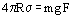where F is a correction factor. If V is the volume of the meniscus, tables give R3/V for the values of R/r.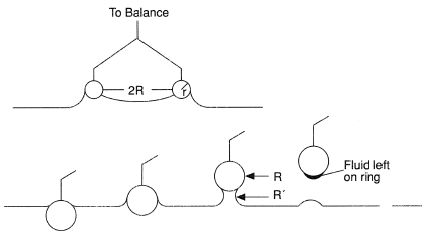Figure 11. du Noüy ring plate method.
The maximum force required to detach the ring is measured together with the density of the liquid and the radii of the ring and wire.
A zero contact angle is necessary, vibrahe surface. The static Wilhelmy plate method is tions must be avoided and the ring must be accurately parallel to tmore reliable.

### The Wilhelmy plate

May be used as a detachment method in which case a thin plate dipping in a fluid is gradually raised and the maximum pull at the point of detachment measured, as shown in Figure 12.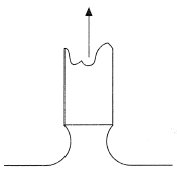Figure 12. Wilhelmy plate method.
For Θ = 0, maximum pull = 2σ (l + t), where l is the length of the plate and t its thickness.
The accuracy of the method is about 0.1%.

### Static Methods

In this method the plate is not detached. If W is the weight of the plate and W’ its weight on immersion, then
(36)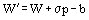where p is the perimeter of plate (p = 2(1 + t)) and b is buoyancy correction, b is 0 if the plate is immersed as shown in Figure 13. The weight, w, of the plate may be offset if an electrobalance is used. Hence
(37)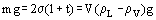Accuracy 0.01 mN/m with care.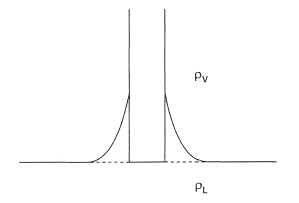Figure 13. Static method.

#### The spinning drop method

This method is particularly suited to the measurement of very low interfacial tensions. A drop of liquid A is placed in a tube filled with liquid B, which has a higher density than A. On spinning the tube as shown in Figure 14 the drop of A moves to the axis of the tube and with increasing velocity of rotation, w, the drop becomes ellipsoidal and finally an elongated cylinder. Rotational velocities of about 20,000 r.p.m. may be used. Consider an element of the cylinder of volume V. The centrifugal force on it is w2r2Δρ/2. Integrating for a cylinder of length l is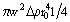, and the interfacial free energy is 2πr0lσ. The total energy, E, is thus
(38)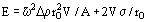since V =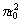l. Putting dE/dr0 = 0 we obtain
(39)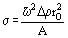Values of interfacial tension as low as 10−3 mNm−1 can be measured readily and accurately. Precision bore tubing must be used and the apparatus constructed with precision. Account must be taken for the lens effect produced by the outer fluid.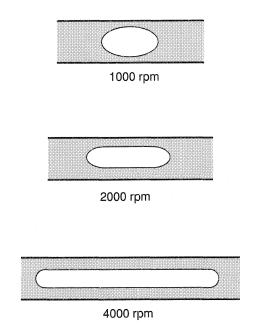Figure 14. Spinning drop method.

### Dynamic Methods

#### Ripples

Kelvin showed that the velocity of ripples on the surface of a liquid is given by
(40)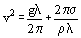where λ is the wavelength of the wave and ρ is the density of the liquid.
Hence,
(41)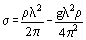Moving or stationary waves may be used. For moving waves stroboscopic methods are used.

Table 3. Choice of method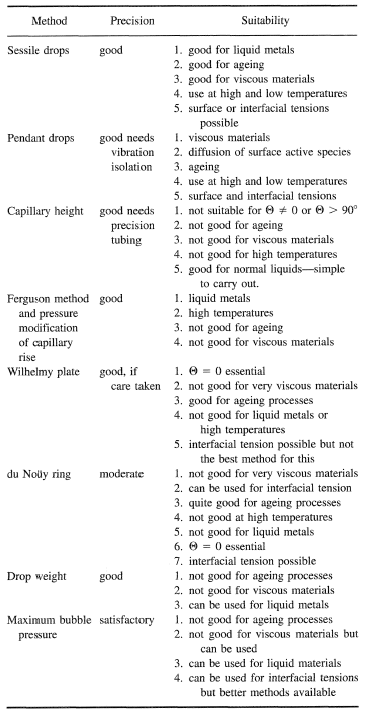#### Oscillating jets

If fluid emerges from a noncircular orifice the jet shows a number of oscillations as illustrated in Figure 15. Surface tension counteracts the noncircular shape of the jet, tending to bring it back to a circular crosssection. The momentum causes the jet to overshoot the circular form and so the oscillations are formed. The rate of flow is measured and hence the age of the surface at any point along the jet. The wavelength is measured photographically and from the variation of the wavelength along the jet, the surface tension corresponding to various ages may be determined. Surface ages of order of 2 m sec may be measured by this method.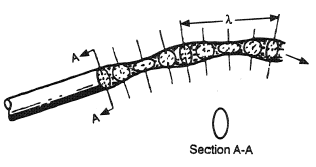Figure 15. Oscillating jet.
For more details on dynamic methods see original papers [Fordhan (1948) & Rideal & Sutherland (1952)].
Comprehensive tables of values for surface tension can be found in Rosen (1972) and Quayle (1953).
##### General References
Adamson, A. W., Physical Chemistry of Surfaces, 3rd end., John Wiley and Sons, New York.
Davies, J. T. and Rideal, E. K. (1961) lnterfacial Phenomena, Academic Press, New York.
Ross, S. and Morrison, I. (1988) Colloidal Systems and Interfaces, John Wiley.